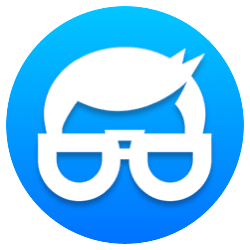## AP Physics 1 - The Complete Guide 2023 (learn Physics from scratch)

Dive in and learn AP Physics 1 from scratch! Learn Kinematics, Linear and Rotational Dynamics, Energy, Best Practices and way more!

4.8
4.8/5

## 15% of curriculum • 8 hours to complete

Covers the following topics:

• vectors and scalars
• standard units
• Understanding the 5 kinematic variables (∆x, v0, vf, a, and t)
• Understanding the 4 kinematic equations
• Motion graphs
• Problem solving in 1 and 2 dimensions (projectile motion)

## 18% of curriculum • 6 hours to complete

Covers the following topics:

• Applying Newton’s laws in depth
• Common linear forces
• Drawing force body diagrams
• Solving 10 different types force problems

## 7% of curriculum • 2 hours to complete

Covers the following topics:

• Centripetal forces (conceptually)
• Solving centripetal motion problems
• Gravitational forces (conceptually)
• Solving gravitational problems
• Combining gravitational and centripetal forces with orbits and satellites.

## 24% of curriculum • 8 hours to complete

Covers the following topics:

• 4 types of energy in AP Physics 1
• Conservation of energy
• Using conservation of energy to solve problems
• Understanding power
• Other types of energy (bonus)
• Advanced energy problems to challenge you

## 15% of curriculum • 6 hours to complete

Covers the following topics:

• Understanding momentum conceptually
• Types of collisions
• Momentum vs impulse
• Conservation of momentum
• Applying conservation of momentum to solve collision problems
• Using impulse to solve all other problems

## 6% of curriculum • 2 hours to complete

Covers the following topics:

• Harmonic motion (conceptually)
• Springs and pendulums
• Period and frequency
• Applying past energy concepts to solve SHM problems

## 15% of curriculum • 8 hours to complete

Covers the following topics:

• Rotational variables and converting between linear and rotational quantities
• Moment of inertia
• Rotational kinematics
• Rotational forces (Torque)
• Rotational energy
• Rotational momentum

## Engineering Physicist, Professional Physics Coach and InstructorFeeling stumped by Physics?

Physics is hard, I get it! But what if I told you it doesn’t have to be?

As your guide, I aim to…

(1) Demystify complex concepts in easy-to-grasp terms,
(2) Elevate your Physics test scores,
(3) Ignite your passion for Physics.

Hi! I’m Jason. With over 500 students reaping success under my guidance, I believe in results-driven teaching.

Forget note-taking and memorization. Physics is all about…

No fluff, just concise, effective lessons.

Your independent work beyond our sessions will require commitment, BUT the payoff is tremendous.

A stellar Physics grade, a boosted confidence level, and an impressive edge in college applications are all within your reach. Not to mention, your problem-solving skills will be notably sharpened.

Book a session with me and let’s carve out the best path for you.

I assure 100% satisfaction or your money back. I look forward to seeing you soon!

## Starts September 23or check out

## 100% off!